•#### Learning Bayesian Models with R#### Overview of this book

Learning Bayesian Models with RCreditswww.PacktPub.comPrefaceFree Chapter
Introducing the Probability TheoryThe R EnvironmentIntroducing Bayesian InferenceMachine Learning Using Bayesian InferenceBayesian Regression ModelsBayesian Classification ModelsBayesian Models for Unsupervised LearningBayesian Neural NetworksBayesian Modeling at Big Data ScaleIndex## Generalized linear regression

Recall that in linear regression, we assume the following functional form between the dependent variable Y and independent variable X: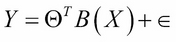Here,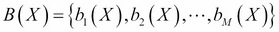is a set of basis functions and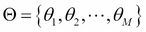is the parameter vector. Usually, it is assumed that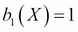, so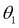represents an intercept or a bias term. Also, it is assumed that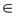is a noise term distributed according to the normal distribution with mean zero and variance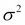. We also showed that this results in the following equation: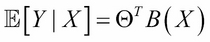One can generalize the preceding equation to incorporate not only the normal distribution for noise but any distribution in the exponential family (reference 1 in the References section of this chapter). This is done by defining the following equation: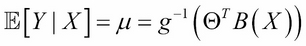Here, g is called a link function. The well-known models, such as logistic regression, log-linear models, Poisson regression, and so on, are special cases of GLM. For example, in the case of ordinary linear regression, the link function would be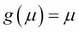. For logistic regression...